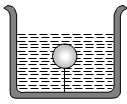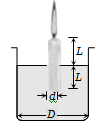A solid sphere of density $\mathrm{\eta }$ ( > 1) times lighter than water is suspended in a water tank by a string tied to its base as shown in fig. If the mass of the sphere is m then the tension in the string is given by(a) $\left(\frac{\mathrm{\eta }-1}{\mathrm{\eta }}\right)\mathrm{mg}$                              (b) $\mathrm{\eta mg}$

(c) $\frac{mg}{\mathrm{\eta }}$                                        (d) $\left(\mathrm{\eta }-1\right)\mathrm{mg}$

Concept Questions :-

Archimedes principle
High Yielding Test Series + Question Bank - NEET 2020

Difficulty Level:

A hollow sphere of volume V is floating on water surface with half immersed in it. What should be the minimum volume of water poured inside the sphere so that the sphere now sinks into the water ?
(a) V/2                        (b) V/3

(c) V/4                        (d) V

Concept Questions :-

Archimedes principle
High Yielding Test Series + Question Bank - NEET 2020

Difficulty Level:

A ball whose density is  falls into water from a height of 9 cm . To what depth does the ball sink
(a) 9 cm                                    (b) 6 cm
(c) 4.5 cm                                 (d) 2.25 cm

Concept Questions :-

Archimedes principle
High Yielding Test Series + Question Bank - NEET 2020

Difficulty Level:

Two solids A and B float in water. It is observed that A floats with $\frac{1}{2}$ of its body immersed in water and B floats with $\frac{1}{4}$ of its volume above the water level. The ratio of the density of A to that of B is
1. 4 : 3                                  2. 2 : 3
3. 3 : 4                                  4. 1 : 2

Concept Questions :-

Archimedes principle
High Yielding Test Series + Question Bank - NEET 2020

Difficulty Level:

A boat carrying steel balls is floating on the surface of water in a tank. If the balls are thrown into the tank one by one, how will it affect the level of water ?

(a) It will remain unchanged

(b) It will rise

(c) It will fall

(d) First it will first rise and then fall

Concept Questions :-

Equation of continuity
High Yielding Test Series + Question Bank - NEET 2020

Difficulty Level:

Two pieces of metal when immersed in a liquid have equal upthrust on them; then

(a) Both pieces must have equal weights

(b) Both pieces must have equal densities

(c) Both pieces must have equal volumes

(d) Both are floating to the same depth

Concept Questions :-

Archimedes principle
High Yielding Test Series + Question Bank - NEET 2020

Difficulty Level:

A candle of diameter d is floating on a liquid in a cylindrical container of diameter D (D>>d) as shown in figure. If it is burning at the rate of 2cm/hour then the top of the candle will(a) Remain at the same height

(b) Fall at the rate of 1 cm/hour

(c) Fall at the rate of  2 cm/hour

(d) Go up the rate of 1cm/hour

Concept Questions :-

Archimedes principle
High Yielding Test Series + Question Bank - NEET 2020

Difficulty Level:

Construction of submarines is based on
(a) Archimedes’ principle                (b) Bernoulli’s theorem
(c) Pascal’s law                              (d) Newton’s laws

Concept Questions :-

Archimedes principle
High Yielding Test Series + Question Bank - NEET 2020

Difficulty Level:

In which one of the following cases will the liquid flow in a pipe be most streamlined  ?

(a) Liquid of high viscosity and high density flowing through a pipe of small radius

(b) Liquid of high viscosity and low density flowing through a pipe of small radius

(c) Liquid of low viscosity and low density flowing through a pipe of large radius

(d) Liquid of low viscosity and high density flowing through a pipe of large radius

Concept Questions :-

Types of flow
High Yielding Test Series + Question Bank - NEET 2020

Difficulty Level:

Two water pipes of diameters 2 cm and 4 cm are connected with the main supply line. The velocity of flow of water in the pipe of 2 cm diameter is

(a) 4 times that in the other pipe

(b) times that in the other pipe

(c) 2 times that in the other pipe

(d) times that in the other pipe

Concept Questions :-

Equation of continuity